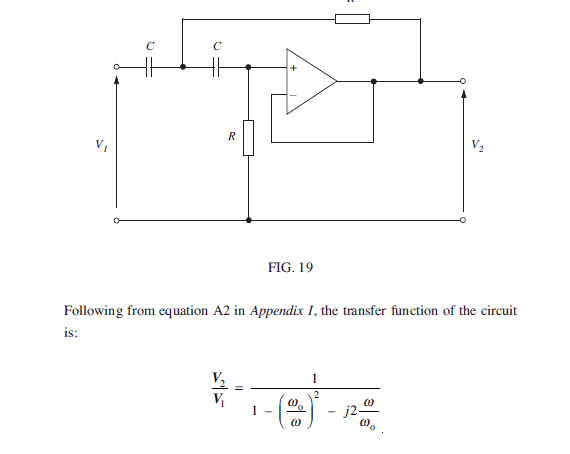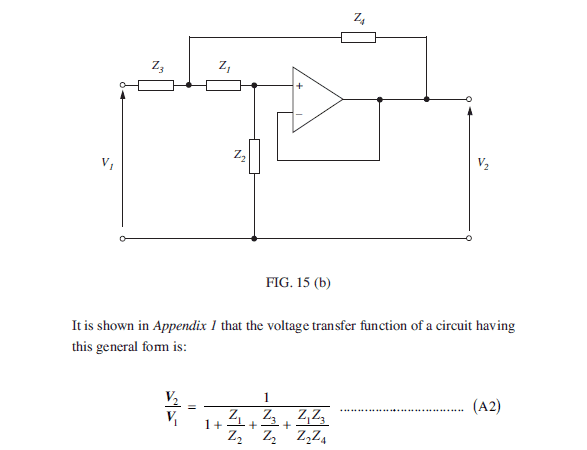# Third order high pass filter

## Homework Statement

Write a report (of approximately 750 words) that discusses and compares
the design of each filter in terms of their performance, circuit topology,
application and any other relevant characteristics. You may for example
attempt to relate the choice of component values to the filters
performance.

## Homework Equations

$$f_c=\frac{1}{2{\pi}RC}$$

## The Attempt at a Solution

The topology of the of the circuit is a first order high pass filter followed by a second order Sallen and key.

As the first part is an RC high pass buffered circuit, I believe the TF can be cascaded (multiplied).

I'm unsure how to work out the corner frequency
Do I work out each part of the circuits Fc?
or is there an overall equation for this circuit

The response of the overall circuit must be to do with the Q factor of the Sallen and key

All help is appreciated
Thanks

#### Attachments

•fig4.JPG
48 KB · Views: 496

rude man
Homework Helper
Gold Member
Analyze the S-K (2nd) section the way you were taught (KVL or KCL). That will give you the transfer function of that section. Then combine with the 1st order high-pass of the 1st section. Then construct Bode plots (frequency and phase asymptotes) for the two concatenated sections.

For plotting the BODE diagram you do not need the transfer function (since all values are given).
However, I dont think that this alone will solve your problem.
For a 750 word document you must do something more.
Here in this forum, we certainly will not solve the whole task - but we can give you some hints.
Therefore:
* Find the GENERAL second order highpass function (books, internet research) - expressed in terms of "pole frequency wp" and "pole quality factor Qp".
* Compare this transfer function (element by element) with the specific transfer function for the second part of your circuit (second order function).
* This gives you the pole parameter wp and Qp for your filter circuit.
* These values can be compared with classical filter tables. This allows you to decide if it is a Butterworth or Chebyshev response or something else.
* In this respect, the known pole frequency of the 1st stage can also be used because - in total - you have a 3rd order filter.
* Finally, you can compare your theoretical findings with a BODE plot for the magnitude of the function.

* These values can be compared with classical filter tables. This allows you to decide if it is a Butterworth or Chebyshev response or something else.
So it is the response of the circuit dictates whether it's a Butterworth or Cherbyshev. Not the circuit design (layout).

the TF for the second order S&K
$$\frac{V_{out}}{V_{in}}=\frac{1}{1-\left( \frac{{\omega}_o}{{\omega}} \right)^2-j2\frac{{\omega}}{{\omega}_o}}$$

"So it is the response of the circuit dictates whether it's a Butterworth or Cherbyshev. Not the circuit design."

The response of the circuit (the transfer function) is the RESULT of your design. Do you think both would be not correlated?

And no - the transfer function is not correct (sign error).
More than that, this is not the GENERAL function - expressed using wp and Qp.
When you are required to write a filter report, it is necessary to know - at least - the terms and the general 2nd order function.

Last edited:This is the TF given in my learning material is this wrong?
More than that, this is not the GENERAL function - expressed using wp and Qp.
I do not know what wp and Qp are abbreviation for. As you can see from above this is the type of format all TF have been derived to by my tutors.

"So it is the response of the circuit dictates whether it's a Butterworth or Cherbyshev. Not the circuit design."

The response of the circuit (the transfer function) is the RESULT of your design. Do you think both would be not correlated?

Obviously the response is due to the TF.

If the circuit has the same basic layout as all these three do it must have the same TF (but different component values) ?
Will they all have the same response type i.e Butterworth or?

View attachment 110010This is the TF given in my learning material is this wrong?
Yes - it is not correct. It is a highpass circuit - and the transfer function magnitude must approach a finit value for very large frequencies.
The last part of your function would cause the magnitude to approach zero for very high frequencies.
View attachment 110010
I do not know what wp and Qp are abbreviation for. As you can see from above this is the type of format all TF have been derived to by my tutors.
So - what is the meaning of "wo" in your expression?
If you investigate filter circuits and corresponding transfer functions, it is a "must" to know about the terms "pole frequency" and "pole Q".
Didnt you hear about these terms? Perhaps you should try to have a short look into a textbook on filter circuits?
Why don`t you try to fill some knowledge gaps by your own?

View attachment 110010
If the circuit has the same basic layout as all these three do it must have the same TF (but different component values) ?
Will they all have the same response type i.e Butterworth or?
[/QUOTE]
Do you really think that the transfer function will not depend on the selected component values?

I see there are lots of names for the same things
The corner frequency or pole frequency
wo = undamped natural frequency in rads-1 = wp = pole frequency in rads-1

Q = quality factor = Qp
Ok after doing more research this is the TF for a High pass Sallen and Key
$$\frac{V_{out}}{V_{in}}= \frac{\left( \frac {j{\omega}} {{\omega}_o} \right)^2}{1+\left( \frac {j{\omega}} {{\omega}_o} \right)^2+\frac{1}{Q} \frac {j{\omega}} {{\omega}_o}}$$
The Frequency is $$\frac{1}{2{\pi} {\sqrt {R_1R_2C_1C_2}}}$$
The Q factor $$Q=\frac {\sqrt {R_1R_2C_1C_2}}{R_1C_2+(C_1+C_2)R_2}$$

So looking at these The Frequency and Q factor are determined by the component values, effecting the response of the TF .

* The transfer function is correct. However, this is not specific to Sallen-Key. It is the general form of a second-order transfer function.
* What means "the frequency is" ? Which frequency ?
* The given expression for the pole-Q is not correct. How did you derive it?

* The transfer function is correct. However, this is not specific to Sallen-Key. It is the general form of a second-order transfer function
This is the TF give in a lot of different places for a S & K and as you said it is just a general second order TF.
So after reading and researching i have tried the Derive the TEUsing $$Z_1=\frac{1}{j{\omega}C_2}~~~~~~~Z_2=R_1~~~~~~~Z_3=\frac{1}{j{\omega}C_1}~~~~~~~Z_4=R_2$$

Inserting into the general form $$\frac{V_2}{V_1} = \frac {1} { 1+ \frac {\frac{1} {j{\omega}C_2} }{R_1} + \frac {\frac{1} {j{\omega}C_1} }{R_1} + \frac {\frac{1} {j{\omega}C_2} }{R_1}\frac {\frac{1} {j{\omega}C_1} }{R_2} }$$

$$\frac{V_2}{V_1} = \frac {1} { 1+ \frac{1} {j{\omega}C_2R_1} + \frac{1} {j{\omega}C_1R_1} + \frac {1} {j{\omega}C_2R_1} \frac{1} {j{\omega}C_1R_2} }$$

$$=\frac{1} { 1+\frac{1} {j{\omega}} \left( \frac{1} {C_2R_1} + \frac{1} {C_1R_1} \right) + \frac{1} {(j{\omega})^2} \left(\frac{1} {C_2R_1C_1R_2} \right) }$$

multiplying by (jω)^2
$$=\frac{(j{\omega})^2} {(j{\omega})^2 + j{\omega} \left(\frac{1} {C_2R_1} + \frac{1} {C_1R_1} \right) + \left(\frac{1} {C_2R_1C_1R_2}\right)}$$
* What means "the frequency is" ? Which frequency ?
By frequency I meant the pole/corner frequency ƒc

By frequency I meant the pole/corner frequency ƒc

Note that the pole frequency is NOT identical to the 3dB-corner frequency (Exception: Butterworth response).
In your post#8 you have the general second-oder function (expressed in wp and Qp).
At the end of your post#10 you have the transfer function which belongs to your circuit.
Now - comparing both functions it is a simple task to find the correct Q expression for your circuit.
Then, you can insert the given parts values to find the actual Qp value for your example.

•topcat123
Thanks
I know that$${{\omega}_O}^2=\frac{1}{\sqrt{R_1R_2C_1C_2}}$$
so the part in brackets must included Q

$$\left(\frac{1}{R_1C_2}+\frac{1}{R_1C_1}\right)$$

Note that the pole frequency is NOT identical to the 3dB-corner frequency (Exception: Butterworth response).[/QUOTE
Can you explain the difference.
I understand each part of the circuit has its own pole frequency, is the corner frequency the overall response of the circuit.

So - the unit of Qp is 1/time? No - that is not correct.

Ok $$\frac{{{\omega}_O}^2}{Q}=\frac{1}{R_1C_1}+\frac{1}{R_1C_2}$$
Giving Q$$Q=\frac{\sqrt{R_1R_2C_1C_2}}{R_1(C_1+C_2)}$$
??